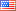##### THE DESCRIPTIVE COMPLEXITY OF THE FAMILY OF BANACH SPACES WITH THE BOUNDED APPROXIMATION PROPERTY
Publication Type
Original research
Authors

We show that the set of all separable Banach spaces that have the bounded approximation property (BAP) is a Borel subset of the set of all closed subspaces of $C(\Delta)$, where $\Delta$ is the Cantor set, equipped with the standard Effros-Borel structure. Also, we prove that if $X$ is a separable Banach space with a norming M-basis $\{e_{i} , e_{i}^{*} \}_{i=1}^{\infty}$ and $E_{u}=\overline{span} \{e_{i};\,\,i \in u\}$ for $u \in \Delta,$ then the set $\{u \in \Delta;\,\,E_{u}\,\,has\,\,a\,\,FDD\}$ is comeager in $\Delta$.

Journal
Title
HOUSTON JOURNAL OF MATHEMATICS
Publisher
University of Houston, Houston, Texas ISSN 0362-1588
Publisher Country
United States of AmericaIndexing
Thomson Reuters
Impact Factor
0.273
Publication Type
Both (Printed and Online)
Volume
43
Year
2017
Pages
395-401